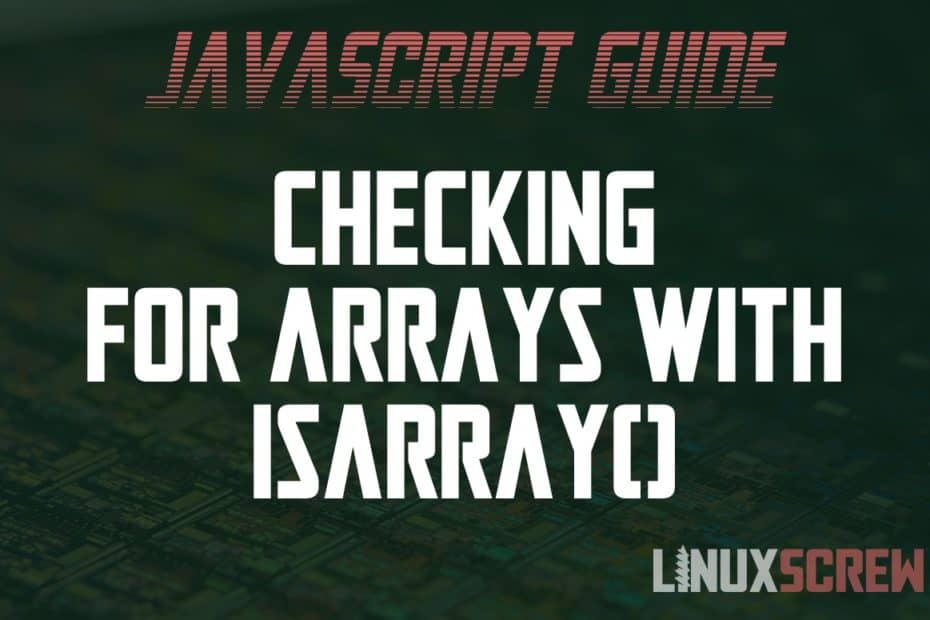# Check if a JavaScript Variable is an Array with isArray() [Examples]

• JavascriptHere’s a short article that explains arrays and how to check if a JavaScript variable is an array using the Array.isArray() method.

Want to check out whether an array contains a value? Find out how here.

## What is An Array?

An array is a type of JavaScript variable that can hold other variables, or references to other variables, in a list at a certain position.

## Declaring an Array in JavaScript

An array is declared in JavaScript the same way as any other variable – by assigning the value to a variable name.

An array is constructed using square brackets ([]) to contain the array values, which are separated by a comma (,) – here it is in code:

`var myArray = ['dog', 'cat', 'pig'];`

Above, an array variable called myArray is declared with the value of an array containing the strings dog cat and pig.

Each element in the array is its own value, contained within the array at a certain position (index).

## Checking for Arrays using Array.isArray()

If you have a variable that could take on any number of values (for example, from reading a file or user input) and you want to check whether or not it is an array – the Array.isArray() method is the tool that does just that.

Here’s how it’s used:

```var myArray = ['dog', 'cat', 'pig'];

Array.isArray(myArray);  // Returns true```

Above, the declared myArray variable is passed to Array.isArray(), which returns a value of true as an array value was found.

Values that are not an array will return false:

```Array.isArray({attribute: 'value'}); // Returns false as it is an object
Array.isArray('hello!'); // Returns false as it is a string
Array.isArray(null);  // Returns false as it is null```

Easy! As boolean values are returned by Array.isArray(), you can use an if statement to quickly check a variable and take the appropriate action if an array is found:

```if(Array.isArray(myArray)){
console.log('An array!);
} else {
console.log('Not an array');
}```

SHARE: